# DAV Class 5 Maths Chapter 7 Worksheet 10 Solutions

The DAV Maths Book Class 5 Solutions and DAV Class 5 Maths Chapter 7 Worksheet 10 Solutions of Multiplication and Division of Decimal Numbers offer comprehensive answers to textbook questions.

## DAV Class 5 Maths Ch 7 Worksheet 10 Solutions

Question 1.
Find the quotient.
(a) 6 ÷ 0.2
Solution: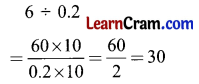(b) 15 ÷ 0.05
Solution: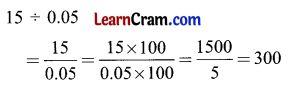(c) 64 ÷ 0.32
Solution: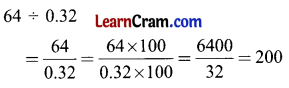(d) 822 ÷ 1.644
Solution: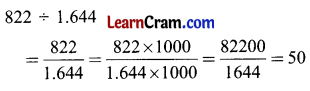(e) 31 ÷ 0.5
Solution: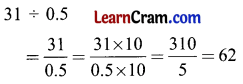(f) 81 ÷ 0.27
Solution:(g) 13 ÷ 0.13
Solution:(h) 225 ÷ 7.5
Solution: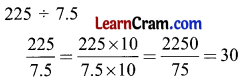(i) 100 ÷ 2.5
Solution:(j) 112 ÷ 1.6
Solution: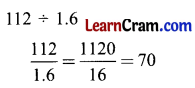DAV Class 5 Maths Chapter 7 Worksheet 10 Notes

Division of a Whole Number by a Decimal Number

Example 1.
Divide 9 by 0.3
Solution:
9 ÷ 0.3
= $$\frac{1}{2}$$ (Division expressed as a fraction)
= $$\frac{9 \times 10}{0.3 \times 10}$$ (Divisor has one decimal place. So, multiply both numerator and denominator by 10)
= $$\frac{90}{3}$$
= 30Example 2.
Divide 54 by 0.03
Solution:
54 ÷ 0.03
= $$\frac{54}{0.03}$$
= $$\frac{54 \times 100}{0.03 \times 100}$$
= $$\frac{5400}{3}$$
= 1800